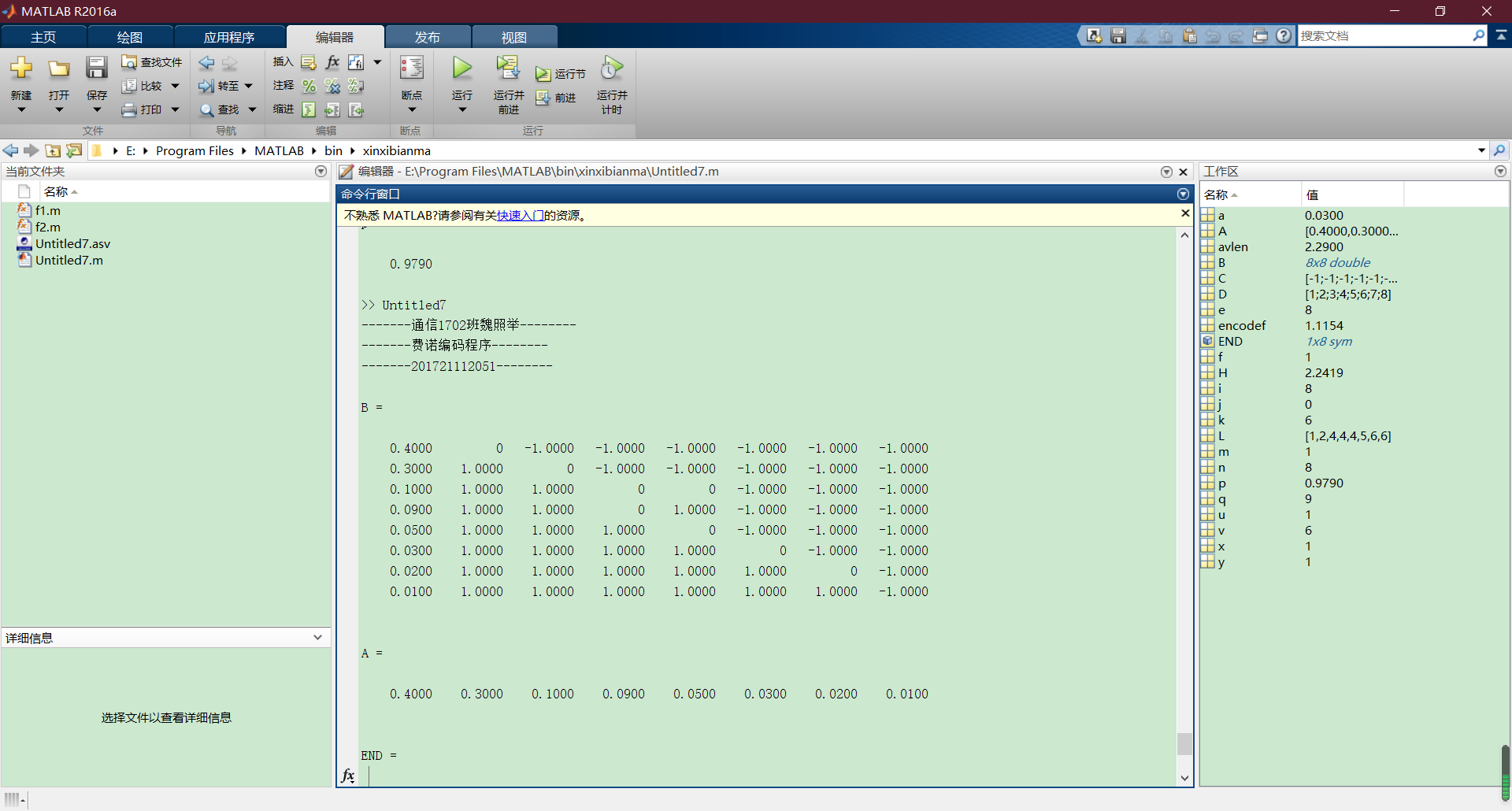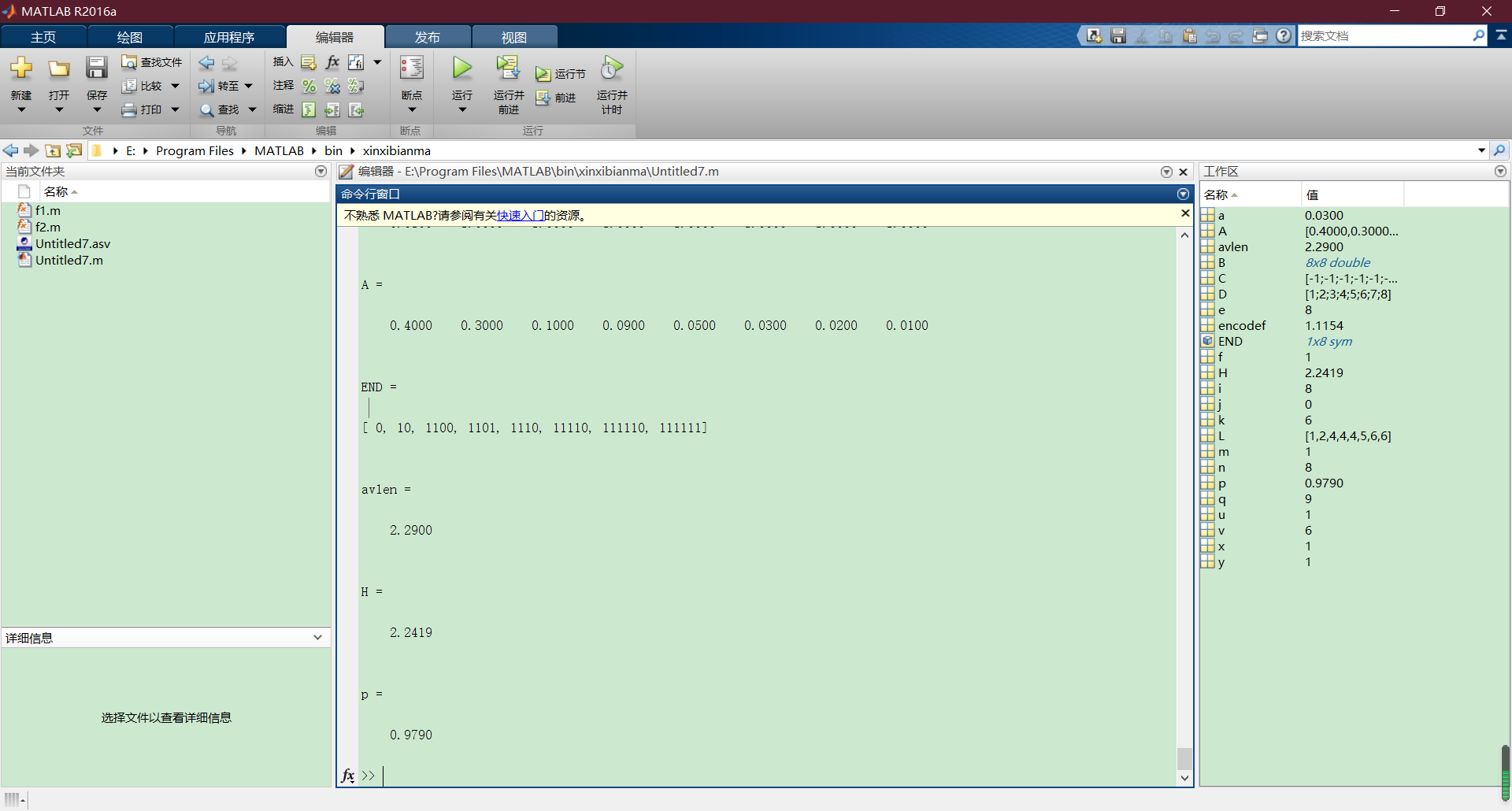• 题目基于 MATLAB 的通信系统仿真 信道编码对通信系统性能的影响 专业 通信工程 姓名崔校通 ...摘要简述信道编码理论详细说明分组码的编译原理实现方法及检错纠错能 力 MATLAB 仿真有无信道编码条件下对通信系统性能
• 用于无线通信的信道编码用matlab编写的模块用于无线通信的信道编码用matlab编写的模块用于无线通信的信道编码用matlab编写的模块用于无线通信的信道编码用matlab编写的模块
• 实现汉明编码，对matlab实现汉明编码有重要意义，可用于图像，音频中嵌入信息，减小误码率。
• matlab USTC 信息论 A 简单信道编码定理实现 channel coding 课程作业codes 课程作业codes 课程作业codes
• 各种信道编码Matlab和FPGA的实现，包括MATLAB,FPGA工程完整代码
• 通过MATLAB编程实现固定二元对称信道（BSC），并与MATLAB内部的函数bsc进行对比，验证结果符合BSC信道特点。
• #信道编码与交织 ##差错控制方式 在数字同通信控制系统中，利用纠错码或检错码进行差错控制的方式有三种：前向纠错、纠错重发、和混合纠错，它们的系统构成如下图所示： ###1、前向纠错控制方式 前向纠错方式（FEC...
信道编码与交织
差错控制方式

在数字同通信控制系统中，利用纠错码或检错码进行差错控制的方式有三种：前向纠错、纠错重发、和混合纠错，它们的系统构成如下图所示：1、前向纠错控制方式

前向纠错方式（FEC）是指发端信息经纠错编码后实现传送，接收端通过纠错编码通过纠错译码器不仅能够自动地发现错误，而且能够自动地纠正接受码传输中的错误。优点是不需要反馈信道，能进行一个用户对多个用户的同播通信，译码器实时性较好。缺点是译码设备比较复杂。应用领域：语音、图像处理，计算机存储系统、磁盘光盘、激光唱机等。
2、检错重发控制方式

检错重发控制方式也称为反馈重发（ARQ）。发送端发出能够发现（检测）错误的码，接收端收到通过信道传来的码后，在译码器根据该码的编码规则，判决收到的码序列中有无错误产生，如果发现错误，则通过反向信道把判决结果反馈回发送端。优点是编译码设备简单，在同样冗余度下检错码的检错能力比纠错码的纠错能力要高得多。缺点是需要一条反馈信道来传输反馈信息，并要求发送和接收端装备有大容量的存储器以及复杂的控制设备。应用领域：计算机局域网、分组交换网、7号信令网等。
3、混合纠错控制方式

混合纠错控制方式（HEC）是前向纠错（FEC）和反馈重发（ARQ）的结合，发送端发送的码元兼有检错和纠错的能力。优点：这种控制方式具有自动纠错和检错重发的优点，并可达到较低的误码率，应用领域也非常广泛。
纠错码的分类
1、线性码与非线性码

根据纠错码各码组信息和监督元的函数关系，可分为线性码和非线性码。如果函数关系为线性的，即满足一组线性方程式，则称为线性码，否则称为非线性码。
2、分组码与卷积码

根据码组中监督码元与信息码元相互的关联的长度，可分为分组码和卷积码。分组码的各码元仅与本组的信息元有关；卷积码中的码元不仅与本组的信息码元相关，而且还与前面若干组的信息码元有关。

分组码把信息序列以k个码元分组，通过编码器将每组的k元信息按一定的规律产生r个多余码元（称为校验元或监督元）输出长为n=k+r的一个码字（码组）。分组码用（n，k）表示，n表示码长，k代表信息位的数目。

卷积码将信息序列以

k

0

k_0

个码元分段，通过编码器输出长为为

n

0

n_0

的一段码组。但是该码的

n

0

n_0

-

k

0

k_0

个校验元不仅与本段的信息源有关，而且也与其前m_0段的信息源有关，故卷积码用（

n

0

n_0

，

k

0

k_0

，

m

0

m_0

）表示。
3、检错码与纠错码

根据码的用途，可分为检错码和纠错码。检错码以检错为目的，不一定能纠错；而纠错码以纠错为目的，一定能检错。
4、Hamming码、循环码、BCH码、RS码、CRC校验码

Hamming码具有的共同特点是：

（n,k)=(

2

m

2^m

-1,

2

m

2^m

-1-m） 式中，m是大于等于3的正整数。

MATLAB提供了生成Hamming码的函数hammgen，以及用Hamming码编码、解码的code和decode函数。

1、h = hammgen（m）

h = hammgen（m）产生一个

m

×

n

m\times n

的Hamming校验矩阵h，其中，n=

2

m

2^m

-1.

2、[h.g]=hammgen(m)

[h.g]=hammgen(m)产生一个

m

×

n

m\times n

的Hamming校验矩阵h和与h相对应的生成矩阵g。其中，n=

2

m

2^m

-1。h = [I P], I是一个

m

×

m

m\times m

的单位矩阵。而g=[p I]，其中，I是一个

(

n

−

m

)

×

(

n

−

m

)

(n-m)\times (n-m)

的单位矩阵。
Himming编码案例： 1）仿真(7,4)Hamming码的编码及硬判决译码过程。 2）仿真未编码和进行（7,4）Hamming码的编码的QPSK调制通过AWGN信道后的误比特性能比较。 程序代码如下所示：
%仿真（7,4）Hamming码的编码及硬判决译码过程
clear all
N = 10;          %信息比特行数
n = 7;           %Hamming码组长度n=2^m-1
m = 3;           %监督位长度
[H,G] = hammgen(m);            %产生一个(n,n-m)Hamming码的校验矩阵和生成矩阵
x = randi([0,1],N,n-m);        %产生比特数据
y = mod(x*G,2);                %Hamming编码
y1 = mod(y+randerr(N,n),2);    %在每一个编码码组中引入一个随机比特错误
mat1 = eye(n);                 %生成n*n的单位矩阵，其中每一行的1代表错误比特位置
errvec = mat1*H';              %校验结果对应的所有错误矢量
y2 = mod(y1*H',2);             %译码
%根据译码结果对应的错误矢量找出错误比特位置，并纠错
for index = 1:N
for index1 = 1:n
if(y2(index,:) == errvec(index1,:))
y1(index,:) = mod(y1(index,:)+mat1(index1,:),2);
end
end
end
x_dec = y1(:,m+1:end);         %恢复原始信息比特
s = find(x ~= x_dec)           %纠错后的信息比特与原始信息比特对比

%仿真未编码和进行（7,4）Hamming码的编码的QPSK调制通过AWGN信道后的误比特性能比较
clear all
N = 100000;               %信息比特行数
M = 4;                    %QPSK调制
n = 7;                    %Hamming编码码组长度
m = 3;                    %Hamming码监督位长度
graycode = [0,1,3,2];     %格雷编码规则

msg = randi([0,1],N,n-m);      %信息比特序列
msg1 = reshape(msg',log2(M),N*(n-m)/log2(M))';    %重塑信息比特序列
msg1_de = bi2de(msg1,'left-msb');                 %信息比特序列转换位十进制形式
msg1 = graycode(msg1_de+1);                       %格雷编码
msg1 = pskmod(msg1,M);                            %4QPSK调制
Eb1 = norm(msg1).^2/(N*(n-m));                    %计算比特能量
msg2 = encode(msg,n,n-m,'hamming/binary');        %Himming编码
msg2 = reshape(msg2',log2(M),N*n/log2(M))';       %重塑编码后序列
msg2 = bi2de(msg2,'left-msb');                    %比特序列转换位十进制形式
msg2 = graycode(msg2+1);                          %格雷编码
msg2 = pskmod(msg2,M);                            %Himming编码数据进行4PSK调制
Eb2 = norm(msg2).^2/(N*(n-m));                    %计算比特能量
EbNo = 0:10;                                      %信噪比
EbNo_lin = 10.^(EbNo/10);                         %转换为线性值
for index = 1:length(EbNo)
sigma1 = sqrt(Eb1/(2*EbNo_lin(index)));       %未编码的噪声标准差
%加入高斯白噪声
rx1 = msg1 + sigma1*(randn(1,length(msg1))+1i*randn(1,length(msg1)));
y1 = pskdemod(rx1,M);                         %未编码4PSK解调
y1_de = graycode(y1+1);                       %未编码的格雷逆映射
[err ber1(index)] = biterr(msg1_de',y1_de,log2(M));   %未编码的误比特率

sigma2 = sqrt(Eb2/(2*EbNo_lin(index)));       %编码的噪声标准差
%加入高斯白噪声
rx2 = msg2 + sigma2*(randn(1,length(msg2))+1i*randn(1,length(msg2)));
y2 = pskdemod(rx2,M);                         %编码4PSK解调
y2_de = graycode(y2+1);                       %编码的格雷逆映射
y2_de = de2bi(y2_de,'left-msb');              %转换为二进制形式
y2_de = reshape(y2_de',n,N)';                 %重塑矩阵
y2_de = decode(y2_de,n,n-m,'hamming/binary'); %译码
[err ber2(index)] = biterr(msg,y2_de);        %编码的误比特率
end
figure();
semilogy(EbNo,ber1,'-ko',EbNo,ber2,'-k*');
legend("未编码",'Hamming(7,4)编码的4PSK在AWGN下的性能');
xlabel(EbNo);
ylabel("误比特率");

卷积码的编译码原理

卷积码又称为连环码，它和分组码有明显的区别。(n,k)线性分组码中，本组r = n-k个监督元仅与本组k个信息元有关，与其他各组无关，即分组码编码器本身是无记忆性的。卷积码则不同，每一个(n,k)码段（也称为子码，通常较短）内的n个码元不仅与该段内信息元有关，而且与前面的m段信息元也相关。通常称m为编码存储。卷积码常用符号(n,k,m)表示。

卷积码的编码器由移位寄存器、模二加法器以及开关电路组成。(2,1,2)卷积码的编码器如下图所示：起始状态，各级移位寄存器清零，即

S

1

S

2

S

3

S_1S_2S_3

为000。

S

1

S_1

等于当前输入数据，而移位寄存器状态

S

2

S

3

S_2S_3

存储以前的数据，输出码字C由下式决定，即：

{

C

1

=

S

1

⨁

S

2

⨁

S

3

C

2

=

S

1

⨁

S

3

\left\{\begin{aligned}C_1&=S_1\bigoplus S_2\bigoplus S_3\\C_2&=S_1\bigoplus S_3\end{aligned}\right.

由于

C

1

C_1

对应的加法输入信号及寄存器

m

1

,

m

2

m_1,m_2

相连，因此，对应的二进制序列是111，对应的八进制序列是7，

C

2

C_2

对应的加法器与输入信号及寄存器

m

2

m_2

相连，因此，对应的二进制序列为101，对应的八进制序列为5，因此，这个卷积编码器生成的多项式是[7 5]。

当输入数据D=[1 1 0 1 0]时，输出码字可以计算出来，具体计算过程参见下表，另外为了保证全部的数据通过寄存器，还必须在数据位后加3个0。

S

1

S_1

11010000

S

2

S

3

S_2S_3

0001111001100000

C

1

C

2

C_1C_2

1101010010110000状态ABDCBCAA

从上述的计算可知，每1位数据，影响m+1个输出子码。称m+1为编码约束度。每一个子码有n个码元，在卷积码中有约束关系的最大码长度则为(m+1)n，称为编码约束长度。（2，1，2）卷积码的编码约束度为3，约束长度为6。
卷积码的编译码案例： 仿真BPSK调制在AWGN信道下分别使用卷积码和未使用卷积码的性能对比，其中，卷积码的约束长度为7，生成多项式为[171,133]，码率为1/2，译码分别采用硬判决译码和软判决译码。 代码如下：
clear all
EbNo = 1:10;             %信噪比范围
N = 100000;              %信息比特个数
M = 2;                   %BPSK调制
L = 7;                   %约束长度
trel = poly2trellis(L,[171,133]);         %卷积码生成多项式
tblen = 6*L;                              %Viterbi译码器回溯深度
msg = randi([0,1],1,N);                   %信息比特序列
msg1 = convenc(msg,trel);                 %卷积编码
x1 = pskmod(msg1,M);                      %BPSK调制
for i = 1:length(EbNo)
%加入高斯白噪声，因为码率为1/2，所以每一个符号的能量要比比特能量少3dB
y = awgn(x1,EbNo(i)-3);
y1 = pskdemod(y,M);                                   %硬判决
y2 = vitdec(y1,trel,tblen,'cont','hard');             %Viterbi译码
[err ber1(i)] = biterr(y2(tblen+1:end),msg(1:end-tblen)); %计算误比特率

y3 = vitdec(real(y),trel,tblen,'cont','unquant');     %软判决
[err ber2(i)] = biterr(y3(tblen+1:end),msg(1:end-tblen)); %计算误比特率
end
ber = berawgn(EbNo,'psk',2,'nodiff');                  %BPSK调制理论误比特率
figure();
semilogy(EbNo,ber,'-kd',EbNo,ber1,'-ko',EbNo,ber2,'-k*');
legend("BPSK理论误比特率",'硬判决的误比特率','软判决的误比特率');
xlabel('EbNo');
ylabel("误比特率");

展开全文数字通信
• 使用MATLAB编写的Turbo编码相关函数，亲测可用，对于学习turbo编码的新手有一定的帮助，注释齐全。
• 有详细的注释，自己写的，可用，对理解turbo编码很有意义！！
• 一本详细介绍纠错编码原理的教材，并且用matlab实现
• 1949年香农在《有噪声时的通信》一文中提出了信道容量的概念和信道编码定理，为信道编码奠定了理论基础。无噪信道编码定理（又称香农第一定理）指出，码字的平均长度只能大于或等于信源的熵。有噪信道编码定理（又...
• 信道编码matlab代码python-guided-filter （快速）Guided Filter的Numpy / Scipy实现。 改编自Kaiming的Matlab代码。 gf.py:guided_filter在一个或三个通道的引导图像（I）上运行过滤器，并在任意数量的通道上过滤...
• 通过完成具体编码算法的程序设计和调试工作，提高编程能力，深刻理解信源编码信道编译码的基本思想和目的，掌握编码的基本原理与编码过程，增强逻辑思维能力，培养和提高自学能力以及综合运用所学理论知识去分析...
一、简述
《信息论与编码》是一门理论与实践密切结合的课程,课程设计是其实践性教学环节之一，同时也是对课堂所学理论知识的巩固和补充。其主要目的是加深对理论知识的理解，掌握查阅有关资料的技能， 提高实践技能，培养独立分析问题、解决问题及实际应用的能力。 通过完成具体编码算法的程序设计和调试工作，提高编程能力，深刻理解信源编码、信道编译码的基本思想和目的，掌握编码的基本原理与编码过程，增强逻辑思维能力，培养和提高自学能力以及综合运用所学理论知识去分析解决实际问题的能力，逐步熟悉开展科学实践的程序和方法。
二、费诺编码的分析与实现
（一）设计原理
1.编码原理 早期的数据压缩来自于人们对概率的了解。当对文字信息进行编码时，如果出现概率较高的字幕赋予较短的编码,为出现概率较低的字母赋予较长的编码,平均编码长度就能缩短不少。著名的Morse 电码就是一个范例。信息论之父CE Shannon曾指出，任何信息都存在冗余，冗余大小与信息中每个符号出现概率(不确定性)有关。他所提出的无失真信源编码定理奠定了数据压缩的理论基础。数据压缩的目的就是要消除冗余，信息论是运用概率论与数理统计的方法研究信息、信源熵、通信系统、数据传输、密码学、数据压缩等问题的应用数学学科。 从DVD到个人电脑，从卫星通信到文件，在我们今天的生活中，信息几乎在每个领域都扮演者重要角色。工程师克劳德香农于1948年奠定了信息论的基础，他指出了通信的极限。基于这一理论产生了数据压缩技术、纠错技术等各个应用技术，这些技术提高了数据传输和存储的效率。信息论将信息的传递作为一种统计现象来考虑，给出了估算通信信道容量的方法。信息传输和信息压缩是信息论研究中的两大领域。这两个方面又由信息传输定理、信源-信道隔离定理相互联系。当然，信息论的重大应用远不止于此。DNA是一种信息存储物质，正事信息论帮助人们解开了生物基因组密码之谜。简单地说信息论包含了生命、宇宙乃至一切。 信息论对现代社会的影响是多方面的。首先，在理论研究方面，信息论所处的地位已远远超出了香农当年所界定的“通信的数学理论”的范畴，得到了不断的扩充和发展,出现了语义信息、语法信息与语用信息等研究与信息的意义有关的学科，以及面向智能研究的全信息理论。如今，信息已成为与物质、能量并列的宇宙中的三个基本要素,世间万物的发展变化可归结为物质、能量和信息的传递和转化过程。另一方面，在科学和技术高度发展的今天，信息的概念也被渗透到许多不同的学科和领域，深入到了社会生活的各个方面,成为可与相对论和量子力学并驾齐驱的新一-代边缘交叉学科的重要组成部分。特别是以信息论、控制论、和系统论为代表的“老三论”以及以普利高津的耗散结构理论，哈肯的协同学和托姆的突变论或艾根的超循环理论为代表的“新三论”的出现，标志着一-代新的边缘交叉学科的兴起。它们的形成和发展对现代科学的研究具有重要的方法论_上的指导意义。
2.信源编码原理 由于信源符号之间存在分布不均匀和相关性，是的信源存在冗余度，信源编码的主要任务就是减少冗余，提高编码效率。具体说，就是针对仙缘输出符号序列的统计特性，寻找–定的方法把信源输出符号序列变换为最短码字序列的方法。信源编码的基本途径有两个:使序列中的各个符号尽可能的相互独立，及解除相关性;使编码中各个符号出现的概率尽可能地相等，即概率均匀化。 信源编码的基础是信息论中的两个编码定理:无失真编码定理和限失真编码定理，前者是可逆编码的基础。可逆是指当信源符号转换成代码后，可从代码无失真的恢复原信源符号。当己知信源符号的概率特性时，可计算它的符号熵，这边是每个信源符号所载有的信息量。编码定理不但证明了必定存在一种编码方法，可使代码的平均长度可任意接近但不低于符号熵，而且还阐明达到这木匾的途径，就是使概率与码长匹配。无失真编码或可逆编码只适用与离散信源。对于连续信源，编成代码后就无法无失真地恢复原来的连续值，因为后者的取值可有无限多个。此时只能根据率失真编码定理在失真受限制的情况下进行限失真编码。信源编码定理出现后，编码定理趋于合理化。关于离散信源编码，从无失真编码定理出发。 若要所编的码是惟一-可译码.不但要求编码时不同的信源符号变换成不同的码字，而且必须要求任意有限长的信源序列所对应的码符号序列各不相同，即要求码的任意有限长N次扩展码都是非奇异码。因为只有任意有限长的信源序列 所对应的码符号序列各不相同，才能把该码符号序列惟一地分割成一个个对应的信源符号，从而实现惟一一的译码。
3.费诺编码原理 费诺编码就是通过使编码中各个句号出现的概率大致相等，实现概率均匀化，从而减少冗余度，提高编码效率。凡是能载荷一定的信息量， 且码字的平均长度最短，可分离的变长码的码字集合称为最佳变长码。在编N进制码时首先将信源消息符号按其出现的额概率–次又小到大排列开来，并将排列好的心愿符号按概率值分N大组，使N组的概率之和近似相同，并对各组赋予一个N进制码元0、…N-1。 之再针对每一个大组内的心愿符号做如上处理，即再分为概率相同的N组，赋予N进制码元。如此重复，直到每组只剩下一个心愿符号为止。此时每个信源符号所对应的码字即为费诺码。针对同一个心愿， 费诺码比香农码平均码长小，消息出书速率大，编码效率高。费诺编码是一种信源编码，它编码后的费诺码要比香农码的平均码长小，消息传输速率大，编码效率高。但它属于概率匹配编码它不是最佳的编码方法。
4.费诺编码特点 费诺编码是一-种信源编码，它编码后的费诺码要比香农码的平均码长小，消息传输速率大，编码效率高。但它属于概率匹配编码它不是最佳的编码方法。 费诺编码属于概率匹配编码，具有如下特点: (1)概率大，则分解次数少;概率小则分解次数多，这符合最佳编码原则。 (2)码字集合是唯一-的。. (3)分解之后先得码字后得码长。.
（二）设计步骤
1.费诺码编码步骤 (1)将信源消息符号按其出现的概率大小依次排列: p2p…2pn。 (2)将依次排列的信源符号按概率值分为两大组，使两个组的概率之和近似相同，并对各组赋予一一个_二进制码元“0”和“1”。 (3)将每–大组的信源符号再分成两组，使划分后的两个组的概率之和近似相同，并对各组赋予一一个二进制符号“0”和“1”。 (4)如此重复，直至每个组只剩下一一个信源符号为止。 (5)信源符号所对应的码子即为费诺码.
三、用MATLAB实现费诺编码
MATLAB程序代码：
fprintf(’-------通信1702班魏照举--------\n’); fprintf(’-------费诺编码程序--------\n’); fprintf(’-------201721112051--------\n’); A=[0.4,0.3,0.1,0.09,0.05,0.03,0.02,0.01]; A=fliplr(sort(A));%降序排列 [m,n]=size(A); for i=1:n B(i,1)=A(i);%生成B的第1列 end %生成B第2列的元素 a=sum(B(:,1))/2; for k=1:n-1 if abs(sum(B(1:k,1))-a)<=abs(sum(B(1:k+1,1))-a) break; end end for i=1:n%生成B第2列的元素 if i<=k B(i,2)=0; else B(i,2)=1; end end %生成第一次编码的结果 END=B(:,2)’; END=sym(END); %生成第3列及以后几列的各元素 j=3; while (j~=0) p=1; while(p<=n) x=B(p,j-1); for q=p:n if x==-1 break; else if B(q,j-1)x y=1; continue; else y=0; break; end end end if y1 q=q+1; end if qp|q-p1 B(p,j)=-1; else if q-p2 B(p,j)=0; END§=[char(END§),‘0’]; B(q-1,j)=1; END(q-1)=[char(END(q-1)),‘1’]; else a=sum(B(p:q-1,1))/2; for k=p:q-2 if abs(sum(B(p:k,1))-a)<=abs(sum(B(p:k+1,1))-a); break; end end for i=p:q-1 if i<=k B(i,j)=0; END(i)=[char(END(i)),‘0’]; else B(i,j)=1; END(i)=[char(END(i)),‘1’]; end end end end p=q; end C=B(:,j); D=find(C-1); [e,f]=size(D); if e==n j=0; else j=j+1; end end B A END for i=1:n [u,v]=size(char(END(i))); L(i)=v; end avlen=sum(L.*A) H=-sum(A.*log2(A))%输出信息熵 p=H/avlen %输出编码效率
四、实验结果结果图：展开全文• 附件包含离散信道以及各种信道编码matlab仿真 Matlab , 仿真 , 附件
• 利用matlab的communication toolbox实现AWGN信道下采用QPSK调制和卷积码编码，然后接收端采用维特比译码并且采用硬判决的系统最终得到的误码率曲线，并且采用BERtool工具将其与理论值进行比较。维特比译码
• %BPSK调制在AWGN信道下，RS码 clear all SNR=-10:10; N=30000; %消息比特个数 ber1=zeros(1,length(SNR)); n=7; k=5; T=1; %符号周期 fs=2; %每个符号的采样点数 % fc=2; ...

%BPSK调制在AWGN信道下，RS码
clear all

SNR=-10:10;
N=30000;      %消息比特个数
ber1=zeros(1,length(SNR));

n=7;
k=5;
T=1;                        %符号周期
fs=2;                     %每个符号的采样点数
% fc=2;                      %载波频率
ts=1/fs;                    %采样时间间隔
t=0:ts:T-ts;                %时间向量

msg=randi([0,1],1,N);

msg8=bi2ba(msg);%二进制转成八进制
msg81=reshape(msg8,5,length(msg8)/5).'; %待转换的矩阵就生成了
msgGF=gf(msg81,3);
msgrs=rsenc(msgGF,n,k); %(5,7)RS编码成功 7个输入 5个输出
%msgrs的格式为length(msg8)/k行，n列
msgrs1=reshape(msgrs.',1,length(msg8)/k*n);
msgrs2=de2bi(double(msgrs1.x),'left-msb');%十进制转二进制
%msgrs2的格式为length(msg8)/k*n行，3列
msgy=reshape(msgrs2',1,length(msg8)/k*n*3);%待调制信号

x1=pskmod(msgy,2);

for ii=1:length(SNR)

y=awgn(x1,SNR(ii));    %高斯信道
y1=pskdemod(y,2);

[hnum,lnum]=size(y1);
yrsgs8=reshape(y1,3,hnum*lnum/3).';
yrsgs81=bi2de(yrsgs8,'left-msb');
yrsgs81=reshape(yrsgs81,n,length(yrsgs81)/n).';
ygsrsdec=rsdec(gf(yrsgs81,3),n,k);
d1=reshape(ygsrsdec.x',1,N/3);
d2=de2bi(d1,'left-msb').';
ygs=reshape(d2,1,N);

[num,ber1(ii)] = biterr(ygs, msg);
disp(ber1);
end
figure(1)
semilogy(SNR,ber1,'-ro') ;
legend('高斯');
title('RS码 2PSK AWGN')
xlabel('信噪比（dB）')
ylabel('误比特率')


展开全文x
• 基于matlab信道编码，循环码，界面设计简单介绍
• ## MATLAB实现香农编码

万次阅读 多人点赞 2018-06-07 19:33:43
最近有个实验是用MATLAB实现香农编码的，在网上看到了别人写的程序，大部分都不支持手动输入信源，我自己就加上了几行，能够直接输入信源分布，下面是程序：pa=input('请输入信源分布:')k=length(pa); %计算信源符号...
最近有个实验是用MATLAB实现香农编码的，在网上看到了别人写的程序，大部分都不支持手动输入信源，我自己就加上了几行，能够直接输入信源分布，下面是程序：
pa=input('请输入信源分布:')k=length(pa);       %计算信源符号个数if min(pa)<0||max(pa)>1    %判断信源概率值是否介于0到1之间   % disp(['信源分布pa(x)=[',num2str(pa),']']);    disp('概率值必须介于0到1之间，请重新输入信源分布');    returnelseif sum(pa)~=1     %判断信源累加和是否为1  %  disp(['信源分布pa(x)=[',num2str(pa),']']);    disp('概率累加和必须等于1，请重新输入信源分布');    returnelse    for i=1:k-1      %for循环进行降序排列        for n=i+1:k            if (pa(i)<pa(n))                t=pa(i);                pa(i)=pa(n);                pa(n)=t;                end        end    endenddisp('信源分布概率从大到小为：'),disp(pa) y=0;%给y赋初值，用来求概率和 f=0;%给f赋初值，用来得到子程序最大循环次数s=zeros(k,1);   %对求和结果进行矩阵初始化b=zeros(k,1);   %对编码位数矩阵初始化w=zeros(k,1);  %对二进制矩阵初始化for m=1:k;       %进行香农编码    s(m)=y;    y=y+pa(m);    b(m)=ceil(-log2(pa(m)));%求得的自信息量向上取整，得到码字长度    z=zeros(b(m),1);    %对码字矩阵初始化    x=s(m);    f=max(b(m));   %把码字最大长度赋给f，用于进行十进制转二进制    w=dtob(x,f);    %调用子程序将十进制转换为二进制    for r=1:b(m)        z(r)=w(r);    end   disp('输出结果为：');   disp('初始概率'),disp(pa(m))   disp('求和结果'),disp(s(m))   disp('编码位数'),disp(b(m))   disp('最终编码'),disp(z')   endsum0=0;sum1=0;for i=1:k       %使用for循环进行信息熵、平均码长求解    a(i)=-log2(pa(i));  %a(i)表示单个信源的自信息量    K(i)=ceil(a(i));   %K(i)表示对自信息量向上取整    R(i)=pa(i)*K(i);    sum0=sum0+R(i);  %求平均码长    c(i)=a(i)*pa(i);    sum1=sum1+c(i);  %信息熵endK1=sum0;H=sum1;Y=H/K1;   %用Y来表示编码效率disp(['信息熵H(X)=',num2str(H),'(bit/sign)']); disp(['平均码长K=',num2str(K1),'(bit/sign)']);
disp(['编码效率=',num2str(Y)]);
上面这一部分是主程序，下面这个是调用子程序进行十进制转换成二进制的：
function y=dtob(x,f)for i=1:f    temp=x.*2;    if(temp<1)        y(i)=0;        x=temp;    else        x=temp-1;        y(i)=1;    end
end
完整的程序就是这样的了
展开全文• 用matlab对（7，4）循环码的误码率进行了仿真
•FPGA
• 简述信道编码理论，详细说明分组码的编译原理、实现方法及检错纠错能力，用MATLAB仿真有无信道编码条件下对通信系统性能的影响及信道编码在不同信道下对通信系统性能的影响，如AWGN信道和深衰落信道。
• matlab 实现卷积编码在maltab中有现成的函数来实现卷积编码。...我是一个小白，需要使用matlab实现卷积编码所以学了一下，看了一些帖子，写的不是特别直白，不是很适合小白学习，所以尝试着写一下。 卷积...
• 信息工程学院 通信工程系 设计题目基于MATLAB线性分组码 编译码仿真设计 班 级 10通信 班 学 号 姓 名 指导老师 年 11 月 15 日 成绩 摘 要 该系统是63线性分组码编码和译码实现它能够对输入三位信息码进行线性分组...
• matlab实现卷积码编码和解码，viterbi维特比译码...

# 用matlab实现信道编码matlab 订阅